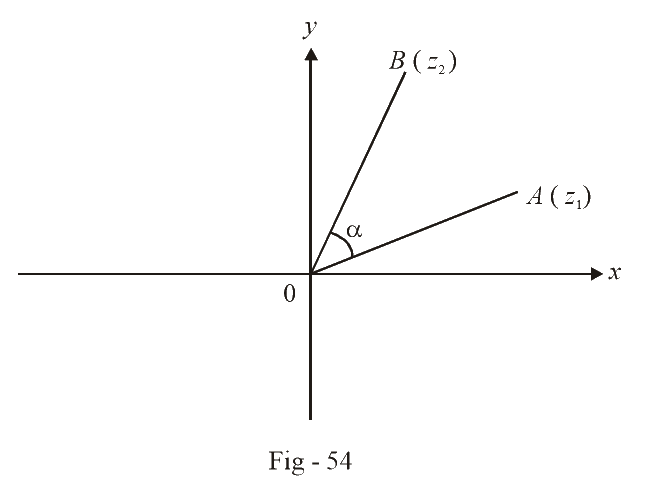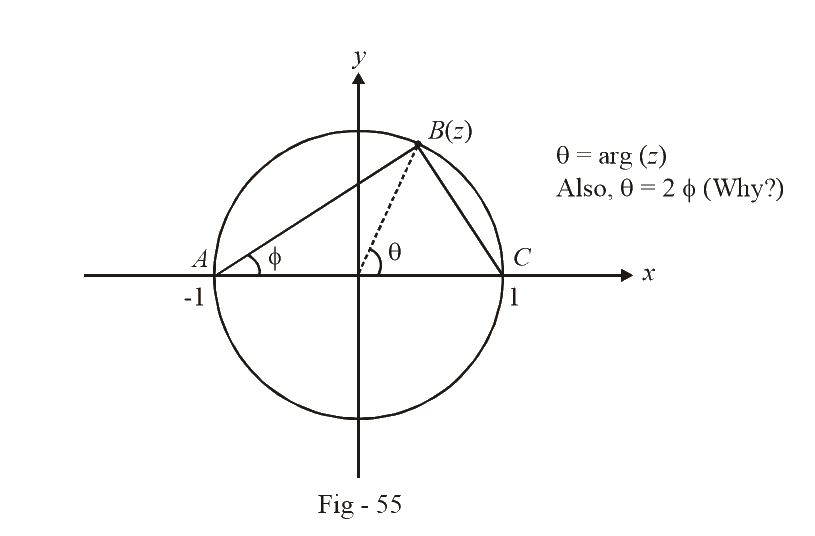# Examples On Rotation Of Complex Numbers

Go back to  'Complex Numbers'

Example-  23

Let $${z_1}$$ and $${z_2}$$ be roots of the equation $${z^2} + pz + q = 0,$$ where the coefficients $$p$$ and $$q$$ may be complex. Let $$A$$ and $$B$$ represent $${z_1}\;and\;{z_2}$$ in the complex plane. If $$\angle AOB = \alpha \ne 0\,\,{\rm{and}}\,\,OA = OB,$$ where $$O$$ is the origin, prove that $${p^2} = 4q\,{\cos ^2}\alpha /2.$$

Solution: The situation described in the question is graphically sketched below:Using rotation $$\left( {OA \to OB} \right),$$ we get:

\begin{align}&\frac{{{z_2}}}{{{z_1}}} = \frac{{OB}}{{OA}}{e^{i\alpha }} = {e^{i\alpha }} = \cos \alpha + i\sin \alpha \ldots (1)\end{align}

Adding 1 to both sides of (1) (it will soon become clear why), we get

\begin{align}&\frac{{{z_2}}}{{{z_1}}} + 1 = 1 + \cos \alpha + i\sin \alpha \\ &\qquad\;\;\;\;= 2{\cos ^2}\alpha /2 + 2i\sin \frac{\alpha }{2}\cos \frac{\alpha }{2}\\ &\qquad\;\;\;\;= 2\cos \frac{\alpha }{2}\left( {\cos \frac{\alpha }{2} + i\sin \frac{\alpha }{2}} \right)\\ &\qquad\;\;\;\;= 2\cos \frac{\alpha }{2}{e^{i\alpha /2}}\ldots (2)\end{align}

Since $${z_1}$$  and $${z_2}$$  are the roots of $${z^2} + pz + q = 0,$$

\begin{align}&\begin{array}{l}{z_1} + {z_2} = - p & & \ldots (3)\\\;\;\;\;{z_1}{z_2} = q\, & & \ldots (4) & \end{array}\end{align}

Substituting (3) in (2) and then squaring, we get

\begin{align}\frac{{{p^2}}}{{z_1^2}} &= 4{\cos ^2}\frac{\alpha }{2}{e^{i\alpha }}\\\,\,\,\,\,\,\,\,\,\,\,\, &=4{\cos ^2}\frac{\alpha }{2}\,\,\,\frac{{{z_2}}}{{{z_1}}}\,\,\, \,\left({{\text{ From}}\left( 1 \right)} \right)\\\Rightarrow \quad {p^2}&= 4{\cos ^2}\frac{\alpha }{2}{z_1}\,{z_2}\\&= 4q{\cos ^2}\alpha /2\,\, \left( {{\text{From }}\left( 4 \right)} \right)\end{align}

Example- 24

If $$\left| z \right| = 1,$$ show that \begin{align}&\frac{{i\left( {1 - z} \right)}}{{1 + z}} = \tan \left( {\frac{{\arg \left( z \right)}}{2}} \right)\end{align}

Solution: We will solve this using both an analytical and a geometrical approach.

ANALYTICAL APPROACH:

Let $$z = r{e^{i\theta }} = {e^{i\theta }} \,\,\,\,\,\left( {{\rm{since}}\,r = 1} \right)$$

\begin{align}& \Rightarrow \,\,\,\frac{{i\left( {1 - z} \right)}}{{1 + z}} = \frac{{i\left( {1 - {e^{i\theta }}} \right)}}{{1 + {e^{i\theta }}}}\\\\ &\qquad\qquad\qquad= \,\,\,\frac{{i\left( {1 - \cos \theta - i\sin \theta } \right)}}{{1 + \cos \theta + i\sin \theta }}\\\\ &\qquad\qquad\qquad= \,\,\,\frac{{\sin \theta + i\left( {1 - \cos \theta } \right)}}{{1 + \cos \theta + i\sin \theta }}\\\\ &\qquad\qquad\qquad= \,\,\,\frac{{2\sin \theta }}{{2 + 2\cos \theta }}\,\,\,\,\,\,\,\,\,\,\,\,\,\,\,\,\,\,\,\,\,\,\,\,\,\,\,\,\left( \rm{Rationalization;\;verify\;this\;step} \right)\\\\ &\qquad\qquad\qquad= \,\,\tan \frac{\theta }{2}\\\\ &\qquad\qquad\qquad= \,\,\tan \,\,\left( {\frac{{\arg \left( z \right)}}{2}} \right)\end{align}

GEOMETRICAL APPROACH:

Let $$z$$ lie anywhere on a unit circle centered at the originApplying rotation $$\left( {AB \to BC} \right),$$

\begin{align}&\quad\;\;\;\frac{{z - 1}}{{z + 1}} = \frac{{BC}}{{AB}}{e^{i\pi /2}} = \frac{{BC}}{{AB}}\,\,i\,\, = i\tan \phi \\\\&\Rightarrow \,\,\,\tan \phi = \frac{1}{i}\left( {\frac{{z - 1}}{{z + 1}}} \right) = \frac{{i\left( {1 - z} \right)}}{{1 + z}}\end{align}

Since \begin{align}&\phi = \frac{\theta }{2},\end{align} we get

$\tan \frac{\theta }{2} = \frac{{i\left( {1 - z} \right)}}{{1 + z}}$

which is the desired result.

Example-  25

Let $${A_1},\,\,{A_2}\,....\,{A_n}$$  be the vertices of an $$n$$-sided regular polygon such that

$\frac{1}{{{A_1}{A_2}}} = \frac{1}{{{A_1}{A_3}}} + \frac{1}{{{A_1}{A_4}}}$

Find the value of $$n.$$

Solution: Referring to Fig - 53 we let the vertices be represented by $${z_1},{z_2}......{z_n}.$$ As in example 22,

\begin{align}&\qquad\quad\;\;{z_2} = {z_1}{e^{i2\pi /n}}\\&\qquad\quad\;\;{z_3} = {z_1}{e^{i4\pi /n}}\\\\&\qquad\quad\;\;{z_4} = {z_1}{e^{i6\pi /n}}\\&\Rightarrow \,\,\,{A_1}{A_2} = \left| {{z_1} - {z_1}{e^{i2\pi /n}}} \right| = \left| {{z_1}} \right|\left| {1 - {e^{i2\pi /n}}} \right|\\\\\,\,\,\,\,\,\,\,\,\,\,\,\,\,\,\,\,\,\, &\qquad\qquad\;= \left| {{z_1}} \right|\left| {\left( {1 - \cos \frac{{2\pi }}{n} - i\sin \frac{{2\pi }}{n}} \right)} \right|\\\\\,\,\,\,\,\,\,\,\,\,\,\,\,\,\,\,\,\, &\qquad\qquad\;= \left| {{z_1}} \right|\left| {\left( {2{{\sin }^2}\frac{\pi }{n} - 2i\sin \frac{\pi }{n}\cos \frac{\pi }{n}} \right)} \right|\\\\\,\,\,\,\,\,\,\,\,\,\,\,\,\,\,\,\,\, &\qquad\qquad\;= 2\left| {{z_1}} \right|\sin \frac{\pi }{n}\end{align}

Similarly,

\begin{align}&{A_1}{A_3} = \left| {{z_1} - {z_1}{e^{i4\pi /n}}} \right| = 2\left| {{z_1}} \right|\sin \frac{{2\pi }}{n}\\&{A_1}{A_4} = \left| {{z_1} - {z_1}{e^{i6\pi /n}}} \right| = 2\left| {{z_1}} \right|\sin \frac{{3\pi }}{n}\end{align}

Substituting $${A_1}{A_2},\,\,{A_1}{A_3}\,\,{\rm{and}}\,\,{A_1}{A_4}$$  for in the relation given in the question, we get

\begin{align}&\;\;\;\quad\frac{1}{{\sin \frac{\pi }{n}}} = \frac{1}{{\sin \frac{{2\pi }}{n}}} + \frac{1}{{\sin \frac{{3\pi }}{n}}}\\ &\Rightarrow \sin \frac{{2\pi }}{n}\sin \frac{{3\pi }}{n} = \sin \frac{\pi }{n}\sin \frac{{2\pi }}{n} + \sin \frac{\pi }{n}\sin \frac{{3\pi }}{n}\end{align}

Writing \begin{align}&\sin \frac{{2\pi }}{n}\end{align} as \begin{align}&2\sin \frac{\pi }{n}\,\,\cos \,\,\frac{\pi }{n}\end{align} and cancelling out \begin{align}&\text{sin} \frac{\pi }{n}\end{align} from both sides, we get

\begin{align}&\qquad2\cos \frac{\pi }{n}\sin \frac{{3\pi }}{n} = \sin \frac{{2\pi }}{n} + \sin \frac{{3\pi }}{n}\\ &\Rightarrow \,\,\,\sin \frac{{4\pi }}{n} = \sin \frac{{3\pi }}{n}\\ &\Rightarrow \,\,\,\frac{{4\pi }}{n} = \pi - \frac{{3\pi }}{n}\,\,\,\,\,\,\,\,\,\,\,\,\,\,\,\,\,\,\,\,\,\,\,\,\,\,\,\,\,\,\,\left( \text{why?} \right)\\ &\Rightarrow \,\,\,n = 7\end{align}

## TRY YOURSELF - VII

Q. 1  $$ABCD$$ are the vertices of a square in the anticlockwise sense, where $$A$$ and $$B$$ are the points $$1 + 2i$$ and $$6 - 2i$$  respectively. Find the points $$C$$ and $$D.$$

Q. 2  Let $$A({z_1}),\;B({z_2})\;{\rm{and}}\;C({z_3})$$  be the vertices of triangle $$ABC.$$ If $$z_1^2 + 2z_2^2 + z_3^2 = 2{z_2}({z_1} + {z_3})$$ , what can we say about $$\Delta ABC$$ ?

Q. 3  Let $$O(0),\;A({z_1})\;{\rm{and}}\;B({z_2})$$  be the vertices of $$\Delta OAB$$ such that $$z_1^2 + {z_1}{z_2} + z_2^2 = 0$$ . What can be said about $$\Delta OAB$$ ?

Q. 4  If $${z_1},\;{z_2}$$  be the roots of the equation $${z^2} + az + b = 0$$, then find the condition such that the origin and points represented by $${z_1}$$  and $${z_2}$$ form an equilateral triangle.

Learn from the best math teachers and top your exams

• Live one on one classroom and doubt clearing
• Practice worksheets in and after class for conceptual clarity
• Personalized curriculum to keep up with school